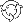The Theory \ Details \ analytical model

# Analytical Model

## Analytical Model of Financial Market

Warning! Reading of the subsequent text assumes basic knowledge of probability theory.

The Analytical Model of Financial Market (or simply Analytical Model), utilized in SmartFolio, is based on Multidimensional Geometric Brownian Motion – the most common class of stochastic processes used in mathematical finance to model the dynamics of prices.

Consider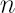risky assets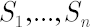available for the investments. Assume also that the investor has access to some
riskless asset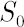, which yields continuously compounded rate of return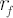that will be referred to as the riskless rate or risk-free rate. An investor expresses prices of assetsin units of asset.
Discounted price of asset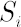at timeis denoted by. Upper asteriskin consequent text denotes transposition operation.

The main assumption of analytical model is given by the following system of stochastic differential equations, which describe evolution of discounted prices of assets: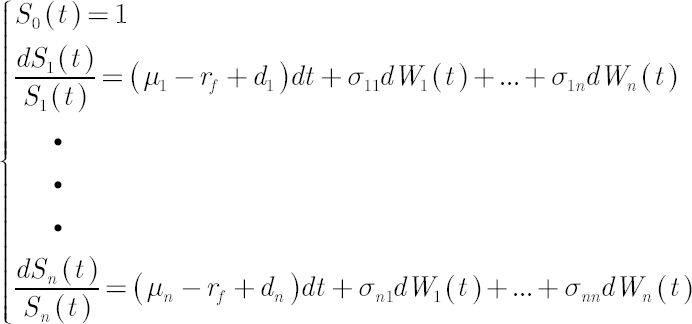In the above expression drift vector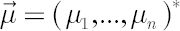(further referred to as the Mu vector), the vector of
continuously compounded
dividend yields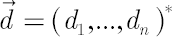and volatility matrix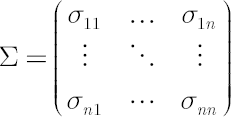consist of constant values, while elements of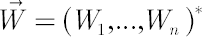represent independent Wiener processes.

Definition. For the sake of convenience the vector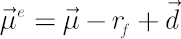, will be further referred to as the Excess Mu vector.
Definition. Matrix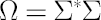is called Covariance Matrix.

Note.
Readers, who are not familiar with stochastic differential equations in continuous time, may interpret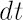as very short time range,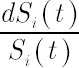as
simple return in-th asset over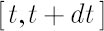and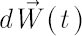as
normally distributed random vector, whose elements are independent of each other (and of components of
other vectors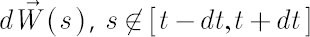), have zero mean and variance.

Denote by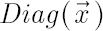diagonal matrix with elements of vectorat the main diagonal. At the same time for diagonal matrix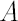denote column vector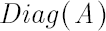, whose elements are equal to diagonal elements of.
In these notations the above system of stochastic differential equations reduces to:A simple solution is presented below: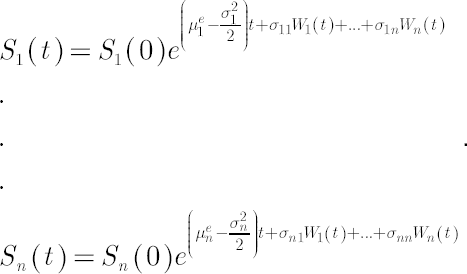where components of the volatility vector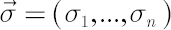are calculated by means of the formula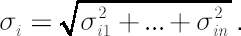Using compact notation, this solution is written as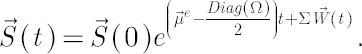Definition. Components of vector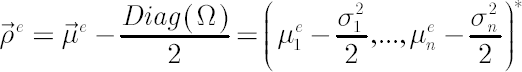are called Expected Excess Growth Rates.

The above definition arises from the fact that for anywhere symbol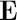denotes mathematical expectation.
On the other hand, it can be shown thatNote. Elements of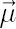are also called Expected Instantaneous Rates of Return, reflecting the notion of expected simple rate of return over an infinitesimally short period of time. Indeed, it is easy to check that for
anythe following chain of equalities holds: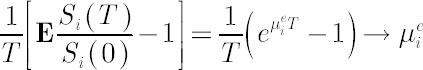when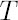approaches zero.